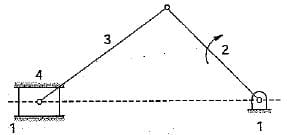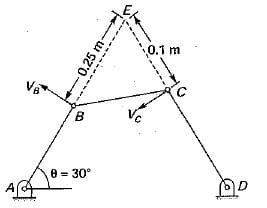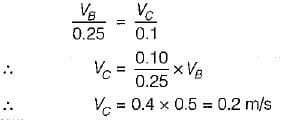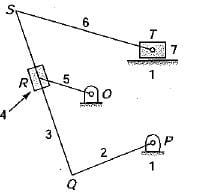Test: Velocity & Acceleration Analysis - 1

# Test: Velocity & Acceleration Analysis - 1

Test Description

## 10 Questions MCQ Test Theory of Machines (TOM) | Test: Velocity & Acceleration Analysis - 1

Test: Velocity & Acceleration Analysis - 1 for Mechanical Engineering 2022 is part of Theory of Machines (TOM) preparation. The Test: Velocity & Acceleration Analysis - 1 questions and answers have been prepared according to the Mechanical Engineering exam syllabus.The Test: Velocity & Acceleration Analysis - 1 MCQs are made for Mechanical Engineering 2022 Exam. Find important definitions, questions, notes, meanings, examples, exercises, MCQs and online tests for Test: Velocity & Acceleration Analysis - 1 below.
Solutions of Test: Velocity & Acceleration Analysis - 1 questions in English are available as part of our Theory of Machines (TOM) for Mechanical Engineering & Test: Velocity & Acceleration Analysis - 1 solutions in Hindi for Theory of Machines (TOM) course. Download more important topics, notes, lectures and mock test series for Mechanical Engineering Exam by signing up for free. Attempt Test: Velocity & Acceleration Analysis - 1 | 10 questions in 30 minutes | Mock test for Mechanical Engineering preparation | Free important questions MCQ to study Theory of Machines (TOM) for Mechanical Engineering Exam | Download free PDF with solutions
 1 Crore+ students have signed up on EduRev. Have you?
Test: Velocity & Acceleration Analysis - 1 - Question 1

### The linear velocity of a point B on a link rotating at an angular velocity ω relative to another point A on the same link is

Detailed Solution for Test: Velocity & Acceleration Analysis - 1 - Question 1

velocity = lω

= ω x AB

Test: Velocity & Acceleration Analysis - 1 - Question 2

### According to Kennedy's theorem, the instantaneous centers of three bodies having relative motion lie on a

Detailed Solution for Test: Velocity & Acceleration Analysis - 1 - Question 2

If three plane bodies have relative motion among themselves, their I-center must lie on a straight line, this is known as Kennedy’s theorem.

Test: Velocity & Acceleration Analysis - 1 - Question 3

### The instantaneous center of a slider moving in a curved surface lies

Detailed Solution for Test: Velocity & Acceleration Analysis - 1 - Question 3

In a sliding motion, the I-center lies at infinity in a direction perpendicular to the path of motion of the slider. The sliding motion is equivalent to a rotary motion of the links with the radius of curvature as infinity.

Test: Velocity & Acceleration Analysis - 1 - Question 4

The given figure shows a slider mechanism in which link 1 is fixed. The number of instantaneous centers would beTest: Velocity & Acceleration Analysis - 1 - Question 5

A four bar mechanism ABCD is shown in the given figure. If the linear velocity ‘VB’ of the point ‘B’ is 0.5 m/s, then the linear velocity ‘Vc’ of point ‘C’ will beDetailed Solution for Test: Velocity & Acceleration Analysis - 1 - Question 5

E i s instantaneous center for the link A B and C D and angular velocity for the point B and C would be same about ETest: Velocity & Acceleration Analysis - 1 - Question 6

Examine the figure shown below wherein the numbers indicate the links:Which of the statements given below are correct?
1. I34 is at ∞, perpendicular to QS
2. I45 is at perpendicular to QS
3. I71 is at T
4. I45 is at R

Test: Velocity & Acceleration Analysis - 1 - Question 7

A line drawn through an instantaneous center and perpendicular to the plane of motion is called instantaneous axis. The locus of this axis is known as

Test: Velocity & Acceleration Analysis - 1 - Question 8

The instantaneous center of rotation of a circular disc rolling on a straight path is

Test: Velocity & Acceleration Analysis - 1 - Question 9

The locus of instantaneous center of a moving body relative to a fixed body is known as the

Detailed Solution for Test: Velocity & Acceleration Analysis - 1 - Question 9

A centrode is the locus of the I-center of a plane body relative to another plane body for the range of motion specified or during a finite period of time.
Space centrode (or fixed centrode) is the locus of the I-center of the moving body relative to the fixed body.
Body centrode (or moving centrode) is the locus of the I-center of the fixed body relative to the movable body.

Test: Velocity & Acceleration Analysis - 1 - Question 10

The space centrode of a circular disc rolling on a straight path is

## Theory of Machines (TOM)

95 videos|43 docs|28 tests
 Use Code STAYHOME200 and get INR 200 additional OFF Use Coupon Code
Information about Test: Velocity & Acceleration Analysis - 1 Page
In this test you can find the Exam questions for Test: Velocity & Acceleration Analysis - 1 solved & explained in the simplest way possible. Besides giving Questions and answers for Test: Velocity & Acceleration Analysis - 1, EduRev gives you an ample number of Online tests for practice

## Theory of Machines (TOM)

95 videos|43 docs|28 tests